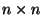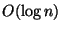Next: Storage and Retrieval Up: Weighted Set Problems Previous: MINIMUM ARRAY PARTITION   Index

### MINIMUM RECTANGLE TILING

• INSTANCE: Anarray A of non-negative numbers, positive integer p.
• SOLUTION: A partition of A into p non-overlapping rectangular subarrays.
• MEASURE: The maximum weight of any rectangle in the partition. The weight of a rectangle is the sum of its elements.

• Good News: Approximable within 2.5 .
• Bad News: Not approximable within 5/4 .
• Comment: The dual problem, where the solution is a tiling where each rectangle has weight at most p, and the objective is to minimize the number of rectangles, is approximable within 2. MAXIMUM RECTANGLE PACKING, the variation in which l weighted axis-parallel rectangles are given in the input, snd the objective is to maximize the sum of weights of p disjoint rectangles is approximable within.

Viggo Kann
2000-03-20